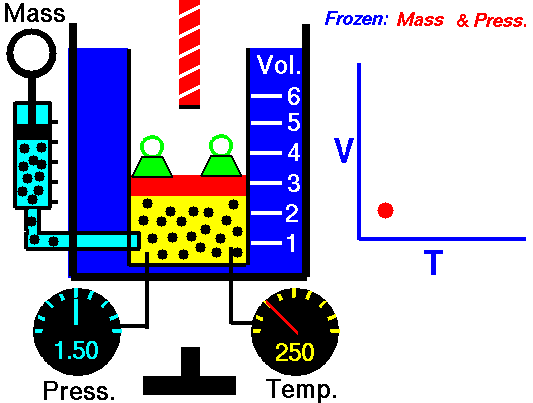# Charles' LawJaques Alaxandre Cesar Charles (1746-1823) studied the compressibility of gases nearly a century after Boyle put forward his Law. In his experiments he observed "At a fixed pressure, the volume of a gas is proportional to the temperature of the gas." The experiment is simple. It uses atmospheric pressure plus the weight of a mass as the fixed pressure.

A graduated cylinder (one with a scale marked on it so you can read the volume) is fitted with a tightly fitted piston.A fixed mass of gas is trapped under the piston head. The equipment is then immersed in a water bath. A mass is placed on top of the piston which results in a pressure on the gas of the weight of that mass and atmospheric pressure.

The gas volume is measured as the temperature of the water bath is increased and V vs T is plotted on a graph. This is continued over a large range of temperatures.

A straight line is obtained with an intercept of -273.15 oC. This is the result whatever the mass of the gas and whatever the initial volume. If we use a scale of temperature that has this -273.15 oC as 0 degrees (the Kelvin scale) we can say that volume is directly proportional to the absolute temperature.

Charles' Law describes the relationship between the volume and temperature of a fixed mass of gas that is held at a fixed pressure . The pressure is caused by the gas molecules bumping into the walls of the container; so, as the temperature decreases the particles have occupy a smaller volume if the pressure is to remain constant.

So the volume occupied by a gas as temperature increases with the temperature of the gas if the pressure is to remain constant. If you increase the one the other must increase by the same factor so that when you divide them you get the same answer. For example, if the temperature is halved, the volume also will be halved. (Note that the 't' in the graphic above should really be 'T'!)

V/T = constant

Taken from the NASA site: